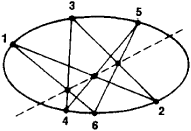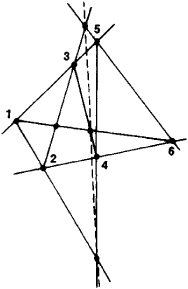Pascal's Theorem

(redirected from Hexagrammum mysticum)

Pascal's theorem

[pa′skalz ‚thir·əm]
(mathematics)
The theorem that when one inscribes a simple hexagon in a conic, the three pairs of opposite sides meet in collinear points.

Pascal’s Theorem

a theorem of geometry asserting that for any hexagon inscribed in a conic section (an ellipse, hyperbola, or parabola) the points of intersection of the three pairs of opposite sides (or of the sides’ extensions) lie on a single line called the Pascal line. The hexagon may be either convex or star-shaped. Figure 1 shows a hexagon whose vertices are successively numbered 1, 2, 3, 4, 5, and 6.Figure 1

Sides that are separated from one another by two sides are considered opposite—that is, the sides 12 and 45, 23 and 56, and 34 and 61. Side 45, for example, is separated from side 12 by sides 23 and 34. The broken line is the Pascal line. If we number the vertices in a different order, that is, if we take a different hexagon, the Pascal line will also be different.

The theorem was established by B. Pascal in 1639. Pappus’ theorem, a special case of Pascal’s theorem for a pair of intersecting lines (a degenerate conic section), has been known since antiquity. This case is shown in Figure 2, where the vertices 1, 3, and 5 lie on one line and the vertices 2, 4, and 6 lie on the otherFigure 2

line; the broken line is the Pascal line. Pascal’s theorem is closely connected with Brianchon’s theorem. These theorems establish important projective properties of conic sections.

REFERENCES

Glagolev, N. A. Proektivnaia geometriia, 2nd ed. Moscow, 1963.
Efimov, N. V. Vysshaia geometriia, 5th ed. Moscow, 1971.
Site: Follow: Share:
Open / Close